# Problem 58259. Easy Sequences 115: Integral involving square root, floor, and round functions

Given a postive real number n, we are asked to evaluate the following integral:where: the symbol "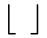" is the floor function,
and "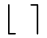" is the round (to the nearest integer) function.
We may rewrite the above function in Matlab as:
>> S = @(n) round(integral(@(x) sqrt(floor(x))-floor(sqrt(x)),0,n));
Therefore, for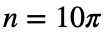, we have:
>> s = S(10*pi)
s =
12
Be careful though, in using the Matlab integral function, as it is only an approximation. For example if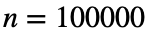:
>> s = S(100000)
Warning: Reached the limit on the maximum number of intervals in use. Approximate bound on error is
8.0e+01. The integral may not exist, or it may be difficult to approximate numerically to the
requested accuracy.
> In integralCalc/iterateScalarValued (line 372)
In integralCalc (line 75)
In integral (line 87)
In @(n)round(integral(@(x)sqrt(floor(x))-floor(sqrt(x)),0,n))
s =
49841
The correct answer is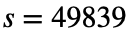. The integral function is off by only 2 units here, but the discrepancy could be even greater for other values of n. integral function can also be quite slow. The challenge is to find an efficient and more accurate algorithm to evaluate the integral.

### Solution Stats

66.67% Correct | 33.33% Incorrect
Last Solution submitted on May 18, 2023

### Community Treasure Hunt

Find the treasures in MATLAB Central and discover how the community can help you!

Start Hunting!